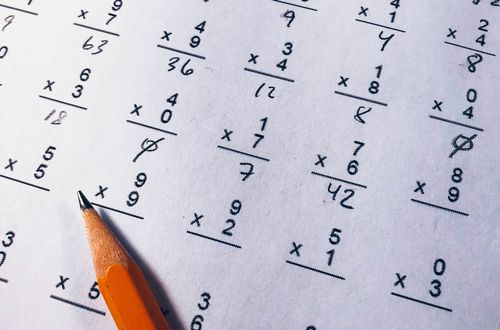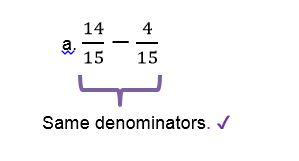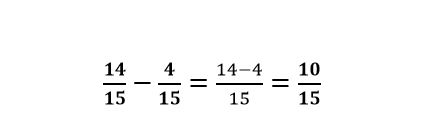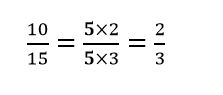# How to calculate fractions with same denominators

Want to find out how to calculate fractions with same denominators? We've provided a step-by-step guide of how to calculate fractions with same denominators to teach you everything you need to know.## Step 1: Add or subtract the numerators

If the denominators of the fractions are the same, add or subtract the numerators right away.

## Step 2: Simplify the fractions

Cancel out common factors to simplify the fractions.

## Examples on calculating fractions with same denominators

**Q1) Perform the indicated operations below. **Since the denominators are the same, we can subtract the numerators directly.Once the numerators are subtracted, see if we can cancel any common factors shared by the numerator and denominator and simplify the difference.#### Join today

The fastest way to practice

Unlock our complete testing platform and improve faster that ever.